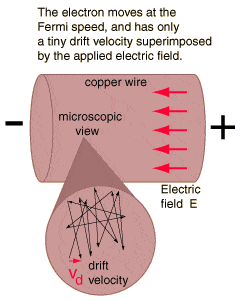# The definition of ohm

Gold Member
The ohm is defined as a resistance between two points of a conductor when a constant potential difference of 1 volt, applied to these points, produces in the conductor a current of 1 ampere, the conductor not being the seat of any electromotive force.

I'm not sure I understand this definition, it's written in a rather confusing manner...instead of saying how much ampere is being stopped from flowing, it tells us how much current is being produced!

That's a little confusing...I thought the definition for the resistance SI unit would be how much of the current is prevented...as in a negative number, maybe.

lorenb
Well, a volt is a POTENTIAL energy. Like say there is a rock on top of a cliff. It has a potential energy, if it were pushed off the edge the potential energy would be converted into kinetic energy.

So, the volt is like a store of charge at one side of a material that wants to go to the side with less charge. (i.e. the rock falling off the cliff to get to a lower energy). If the material slows the charge to move at 1 amp = (1couloumb/sec). The materials resistance will be 1 ohm.

So if the material slowed the charges trying to get to the other side to .1 amps. The materials resistance would be higher.... ( 1/.1 = 10 ohms).

Gold Member
So let me see if I get it right:

1 ohm is 1 amp being stopped per volt, whereas 2 ohm is 2 amp being stopped per volt?...etc etc

lorenb
not being stopped. being allowed through the material. if 1 amp is being allowed through with a 1 volt potential difference. the resistance is 1 ohm. if 2 amps are being allowed through with a potential difference of 1 volt. The resistance is 1volt/2amps. = 1/2 ohm.

The units for OHM = VOLT/AMP

Gold Member
Ah, makes complete sense...so the lower the ohm the lower the resistance right? right. I see now :) thanks.

Gold Member
If you are interested in the http://hyperphysics.phy-astr.gsu.edu/hbase/electric/ohmmic.html" [Broken],check this out.

#### Attachments

Last edited by a moderator: